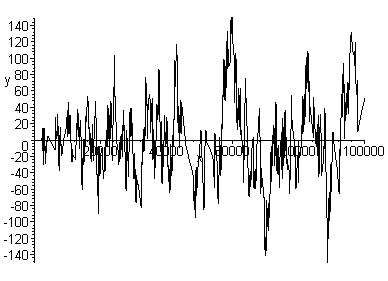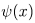an idea to be explored Please watch the animation below before scrolling down to find out more about it. It may take a litte while to load (being an animated GIF of just over 1MB). Once it starts, keep your eyes fixed around the 50,000 mark (the centre into which the animation will zoom). Sitting back some distance from your monitor is recommended.This animated plot zooms into the graph of the function- x (whereis Chebsyhev's logarithmic prime-counting function) from the interval [0,100000] to the central subinterval [49975,50025] (a zoom factor of 2000). The animation is not as smooth as I would have liked, and there are anomalous straight segments which appear in some frames (artefacts of MAPLE's 'adaptive plotting' - please get in touch if you know how to avoid this). However, it suffices to convey the basic idea I would like to explore. The impression I get is of a geometric object with a calculable Hausdorff (or other appropriate fractal) dimension. Clearly, if this is the case, the fractality only holds over a limited range of scales, as we see at the end of the animation when the graph is revealed to be made of discrete 'teeth' - diagonal line segments whose lengths are integer multiples of sqrt(2). If we recall the nature ofas a step function, this is hardly surprising. However, the possibility that a constant fractal dimension might persist over all 'large' scales (i.e. if we were to zoom out indefinitely), but would break down if we zoom back in and approach the unit scale, suggests to me some number theoretical analogue of the Planck length coming into play here. To clarify what I mean by this: If you were watching the animation without any knowledge of what it represented, and if the zoom began from a MUCH LARGER INTERVAL, you might surmise after some years that you were watching an animated plot zooming into a fractal function, and the apparent 'jaggedness' would continue to manifest at all scales, no matter how far in one were to zoom. The sudden halting of the phenomenon at a particular scale, revealing these discrete, 'quantised teeth' might come as something of a surprise, simply because you would have got used to the zoom revealing something whose qualitative 'character' was essentially unchanging. Such a surprise would be somewhat reminiscent of the surprise which accompanied the discovery of the Planck scale in the early 20th century: If we were to hypothetically 'zoom' into the structure of physical space - certain features persist, laws hold, etc. - centuries of belief that physical reality has a continuum-like quality would suggest that this situation should continue indefinitely. But the zoom reaches a certain scale... and THEN suddenly everything becomes discretised, granular, and no information can be extracted from below this (Planck) scale. In both situations, it's as if you were flying towards what appeared to be a vast, distant mountain range, and eventually arrived to discover it was made of Lego bricks.   Laurent Notalle's 'scale relativity' has influenced my thinking, and I have had a lingering intution for some time that it might have some number theoretical application. Notalle argues that to achieve a truly scale invariant physics, we must treat the Planck scale as an invariant under certain groups of scale transformations (much as the speed of light is an invariant with respect to the group of Lorentz transformations). Just as a direct consequence of the invariance of the speed of light is the hyperbolic curvature of spacetime, a direct consequence of the invariance of the Planck scale is that spacetime must have (in a very precise context) an intrinsically fractal nature. This Cantorian-fractal spacetime has been a central feature of the controversial but intriguing approach to the Riemann hypothesis which has been presented by Castro, et.al.   If fratality is a kind of symmetry - symmetry of scale - could the breakdown of fractality near the unit scale (if that indeed is what we are seeing here) be regarded as a type of 'symmetry-breaking'? Would the pole of the Riemann zeta function at s = 1 or the Renormalisation Group possibly have anything to do with this?   Both myself and Carlos Castro who has recently viewed the animation feel that the following paper may be relevant here: "A so-called Renormalization Group (RG) analysis is performed in order to shed some light on why the density of prime numbers in N* decreases like the single power of the inverse naperian logarithm." "...in this note, our aim is to look for the deep reason why the density of primes decreases with the single power of the natural logarithm. We hope that we have been able to shed some light on this fact: the breaking of a symmetry, namely that of scale invariance...is the very factor responsible for this specific decrease. The coincidence of the results obtained is striking when compared to the formulas of the first non-trivial approximation of Quantum ChromoDynamics...But a main common feature emerges: in both cases the two fields are afflicted by the same broken symmetry, that of scale invariance."   Everything about the distribution of primes is logarithmic: The weighting in the von Mangold function and Chebsyhev counting function The sinusoidal functions which the nontrivial Riemann zeros contribute to the explicit formula The familiar form of the Prime Number Theorem average gaps between primes The probabilities of finding primes among integers These things are all clearly inter-related, and it seems that Petermann is trying to get to the root phenomenon, by stepping outside analytic number theory altogether and invoking some fairly basic concepts from renormalisation theory. I only wish I understood more of the physics. The comparison between the Planck length (in Notalle's view) the speed of light c (as understood in conventional relativistic thinking), the numerical unit 1 (in this rather vague speculative note) leads to the question of what group of transformations on the number line preserve the unit. The obvious answer would be the functions x -> xk which, seen through 'logarithmic goggles' look like simple dilations and contractions. So if the invariance of c under the group of Lorentz transformations leads inevitably to the hyperbolic curvature of space time the invariance of the Planck length with respect to the transformations studied by Notalle leads to the inevitable 'fractalisation' of spacetime what does the (seemingly trivial) invariance of 1 under the transformations x -> xk, when looked at from an analogous viewpoint tell us, if anything, about the number system? The parallel with Notalle's relativity is clearly stronger than the parallel with Einstein's relativity, as the transformations we are dealing with here are scaling-related. Hence we might expect any implication to concern some property of the number system (either known and trivial or something altogether new) which was 'of a fractal nature'.   This analogy gives rise to further, rather bizarre, speculation that there might be some related structure as yet unexplored beneath, but somehow 'hidden' below, the unit scale. I seem to recall someone suggesting a number theoretical analogue of the Heisenberg Uncertainty Principle - archived somewhere in my site, but I can't find it now - perhaps I imagined it. The closest thing I can think of at the moment is non-archimedean and p-adic physics, as the existence of the Planck scale violates the Archimedian Principle. My intuition suggests that we should look to the rational numbers, perhaps to Farey series and staircases (with their known fractal properties), if we want to come up with something which continues into ever-smaller ranges of scale the fractality evident in the animation above. In the eventuality that any rigorous mathematics can be invoked to give some meaning to this ill-formed hypothesis, I am fairly certain that p-adic numbers and adeles are going to be somehow involved   This all is unashamedly fuzzy thinking, by the way   Any help at all would be appreciated. This could include debunking the whole idea as nonsense, calculating Hausdorff dimensions (experimentally or analytically), producing smoother, higher-resolution animations, or running experiments over larger intervals. Comments and questions about this idea (or the whole website) are always welcome. Marek Wolf, who has already discovered certain instances of apparent fractality in the distribution of primes, has expressed some scepticism regarding the calculation of a fractal dimension, offering the opinion (15/07): "You will get some number which depends on the method you used." number theory and fractality archive        tutorial        inexplicable secrets of creation        home        contact